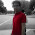Thursday, May 14, 2015

Using Awk to join two files based on several columns

I was reading a thread on stackoverflow and found that this post was very interesting. I will go through the problem and the awk solution. Again, Awk is awesome!

Note:
It looks a bit messy when I copied the ipython notebook directly into Blogger. Ideally, one can write the blog using ipython notebook directly, but it does not work well with Blogger. see here http://blog.fperez.org/2012/09/blogging-with-ipython-notebook.html
I do not want to use other blogging platforms for now. So, just bear it and you can go to here to see the clean ipython notebook. Github now randers ipython notebook.
I created some dummy files.
file_a is a tab-delimited bed file with 6 colums:
In :
```cat file_a.bed
```
```chr1 123 aa b c d
chr1 234 a b c d
chr1 345 aa b c d
chr1 456 a b c d
```
file_b is the file that contain additional infomation, which we want to add to file_a:
In :
```cat file_b.bed
```
```xxxx abcd chr1 123 aa c d e
yyyy defg chr1 345 aa e f g
```
we want to annotate file_a based on the fact that columns 3,4,5 in file_b are the same as columns 1,2,3 in file_a.
To do this, we are going to use Awk associated array. see a link
Let me execute the awk one-liner first and then explain what's going on here:
In :
```awk 'NR==FNR{a[\$3,\$4,\$5]=\$1OFS\$2;next}{\$6=a[\$1,\$2,\$3];print}' OFS='\t' \
file_b.bed file_a.bed
```
```chr1 123 aa b c xxxx abcd
chr1 234 a b c
chr1 345 aa b c yyyy defg
chr1 456 a b c
```
we annotated file_a using file_b. Aka, we added first two columns from file_b to file_a.
There are several things happening here:
we see built-in variables in awk: NR and FNR. NR is the line number of the
current processing line.
when awk read in multiple files, awk NR variable will give the total number of records
relative to all the input file. Awk FNR will give you number of records for each inpu
here
for all the built-in variables in awk.
Let's deomonstrate the difference between NR and FNR:
In :
```awk '{print FILENAME, NR}' file_a.bed file_b.bed
```
```file_a.bed 1
file_a.bed 2
file_a.bed 3
file_a.bed 4
file_b.bed 5
file_b.bed 6
```
FILENAME is another built-in variable for the input file name of awk.
There are 4 lines in file_a and 2 lines in file_b, and NR increments for the total lines.
compare with FNR:
In :
```awk '{print FILENAME, FNR}' file_a.bed file_b.bed
```
```file_a.bed 1
file_a.bed 2
file_a.bed 3
file_a.bed 4
file_b.bed 1
file_b.bed 2
```
Now, awk prints out the line numbers in respect to each file.
From the awk code, we are reading file_b first. NR==FNR means when NR equals to FNR
(this is true only for file_b) do the following: `{a[\$3,\$4,\$5]=\$1OFS\$2;next}`.
We created an associated array named a using columns 3,4,5 in file_b as keys and
the columns 1 and 2: `\$1"\t"\$2` as values. we set OFS="\t" in the end of the command.
next means to proceed for the next line, rather than execute the following { } code block.
when awk reads in the second file (file_a.bed), NR==FNR is not true, awk
exectues the second { } code block: `{\$6=a[\$1,\$2,\$3];print}`
we look up the associated array a we created from file_b.bed using
the first three columns in file_a.bed as keys, and assign column 6 to
the looked-up values and print it out the whole line.
Conclusion: Awk is very powerful in text wrangling. Once get used to the
syntax, you can do fairly complicated formatting in an awk one-liner.
I strongly recommand you to learn it.

1.Any suggestion on how I can print NA or 0 for the 2nd and 4th lines in your example?

chr1 123 aa b c xxxx abcd
chr1 234 a b c 0 0
chr1 345 aa b c yyyy defg
chr1 456 a b c 0 0

1.you may want to use other languages such as R, in dplyr, there is function out_join() can do this.

2.Hey Tommy! You start showing people how great awk is (and I agree with you on this), but then as soon as somebody asks some solution a wee bit more complex, you give up? No!!
Gaurav, what you as for is perfectly doable and even easy in awk, by testing the presence of the entry in the (emulated) multidimensional array a:

awk 'BEGIN { OFS="\t" } ARGIND==1 { a[\$3,\$4,\$5]=\$1 OFS \$2; next } { if ((\$1,\$2,\$3) in a) \$6=a[\$1,\$2,\$3]; else \$6 = "0" OFS "0"; print }' file_b.bed file_a.bed

2.You could use the test ARGIND==1 instead of NR==FNR. The built-in variable ARGIND stores the index of the current file relative to the list of all input files. It's more explicit and probably fractionally faster.